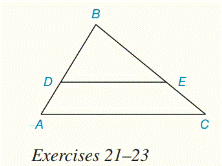Chapter 5.CR, Problem 23CRElementary Geometry For College St...

7th Edition
Alexander + 2 others
ISBN: 9781337614085

Solutions

Chapter
SectionElementary Geometry For College St...

7th Edition
Alexander + 2 others
ISBN: 9781337614085
Textbook Problem

For Review Exercises 21 to 23, D E ¯ | | A C ¯A D = 2 , A B = 10 , B E = 5 , A C = ?

To determine

To find:

The value of BC.

Explanation

Given:

DE¯||AC¯

Calculation:

First to show, ΔDBE similar to ΔABC.

Since, corresponding angles are equal, then

BDE=BAC(DE¯||AC¯)

BED=BCA(DE¯||AC¯)

Here, ΔDBE similar to ΔABC

Let EC=x

Then,

Still sussing out bartleby?

Check out a sample textbook solution.

See a sample solution

The Solution to Your Study Problems

Bartleby provides explanations to thousands of textbook problems written by our experts, many with advanced degrees!

Get Started

Limits at Infinity Find the limit. 8. limx23x4x+5

Precalculus: Mathematics for Calculus (Standalone Book)

53. Demand Graph the first-quadrant portion of the demand function

Mathematical Applications for the Management, Life, and Social Sciences

True or False: n=1(1)nnn+3 converges.

Study Guide for Stewart's Single Variable Calculus: Early Transcendentals, 8th

True or False: The vector function is continuous at t = 3.

Study Guide for Stewart's Multivariable Calculus, 8th